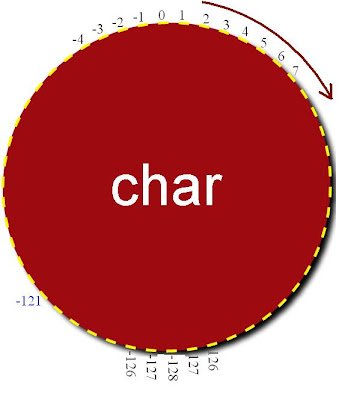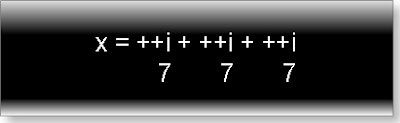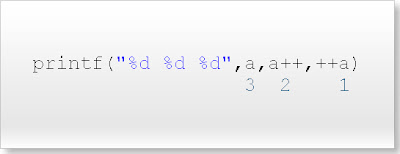## INDEX

Sample c questions and answers with explanation for interview

1.
What will be output if you will compile and execute the following c code?

void main(){
int i=320;
char *ptr=(char *)&i;
printf("%d",*ptr);
}

 (A) 320 (B) 1 (C) 64 (D) Compiler error (E) None of above

Explanation:
As we know size of int data type is two byte while char pointer can pointer one byte at time.
Memory representation of int i=320So char pointer ptr is pointing to only first byte as shown above figure.
*ptr i.e. content of first byte is 01000000 and its decimal value is 64.
Data type tutorial.

2.

What will be output if you will compile and execute the following c code?

#define x 5+2
void main(){
int i;
i=x*x*x;
printf("%d",i);
}

 (A) 343 (B) 27 (C) 133 (D) Compiler error (E) None of above

Explanation:
As we know #define is token pasting preprocessor it only paste the value of micro constant in the program before the actual compilation start. If you will see intermediate file you will find:
test.c 1:
test.c 2: void main(){
test.c 3: int i;
test.c 4: i=5+2*5+2*5+2;
test.c 5: printf("%d",i);
test.c 6: }
test.c 7:
You can absorb #define only pastes the 5+2 in place of x in program. So,
i=5+2*5+2*5+2
=5+10+10+2
=27
Preprocessor tutorial.

3.

What will be output if you will compile and execute the following c code?

void main(){
char c=125;
c=c+10;
printf("%d",c);
}

 (A) 135 (B) +INF (C) -121 (D) -8 (E) Compiler error

Explanation:
As we know char data type shows cyclic properties i.e. if you will increase or decrease the char variables beyond its maximum or minimum value respectively it will repeat same value according to following cyclic order:So,
125+1= 126
125+2= 127
125+3=-128
125+4=-127
125+5=-126
125+6=-125
125+7=-124
125+8=-123
125+9=-122
125+10=-121

4.

What will be output if you will compile and execute the following c code?

void main(){
float a=5.2;
if(a==5.2)
printf("Equal");
else if(a<5.2)
printf("Less than");
else
printf("Greater than");
}

 (A) Equal (B) Less than (C) Greater than (D) Compiler error (E) None of above

Explanation:
5.2 is double constant in c. In c size of double data is 8 byte while a is float variable. Size of float variable is 4 byte.
So double constant 5.2 is stored in memory as:
101.00 11001100 11001100 11001100 11001100 11001100 11001101
Content of variable a will store in the memory as:
101.00110 01100110 01100110
It is clear variable a is less than double constant 5.2
Since 5.2 is recurring float number so it different for float and double. Number likes 4.5, 3.25, 5.0 will store same values in float and double data type.
Note: In memory float and double data is stored in completely different way. If you want to see actual memory representation goes to question number (60) and (61).
Data type tutorial.

5.

What will be output if you will compile and execute the following c code?

void main(){
int i=4,x;
x=++i + ++i + ++i;
printf("%d",x);
}

 (A) 21 (B) 18 (C) 12 (D) Compiler error (E) None of above

Explanation:
In ++a, ++ is pre increment operator. In any mathematical expression pre increment operator first increment the variable up to break point then starts assigning the final value to all variable.
Step 1: Increment the variable I up to break point.Step 2: Start assigning final value 7 to all variable i in the expression.So, i=7+7+7=21
Operator tutorial.

6.

What will be output if you will compile and execute the following c code?

void main(){
int a=2;
if(a==2){
a=~a+2<<1;
printf("%d",a);
}
else{
break;
}
}

 (A) It will print nothing. (B) -3 (C) -2 (D) 1 (E) Compiler error

Explanation:
Keyword break is not part of if-else statement. Hence it will show compiler error: Misplaced break
Control statement tutorial

7.

What will be output if you will compile and execute the following c code?

void main(){
int a=10;
printf("%d %d %d",a,a++,++a);
}

 (A) 12 11 11 (B) 12 10 10 (C) 11 11 12 (D) 10 10 12 (E) Compiler error

Explanation:
In c printf function follows cdecl parameter passing scheme. In this scheme parameter is passed from right to left direction.So first ++a will pass and value of variable will be a=10 then a++ will pass now value variable will be a=10 and at the end a will pass and value of a will be a=12.
Function tutorial.

8.

What will be output if you will compile and execute the following c code?

void main(){
char *str="Hello world";
printf("%d",printf("%s",str));
}

 (A) 11Hello world (B) 10Hello world (C) Hello world10 (D) Hello world11 (E) Compiler error

Explanation:
Return type of printf function is integer and value of this integer is exactly equal to number of character including white space printf function prints. So, printf(“Hello world”) will return 13.

9.

What will be output if you will compile and execute the following c code?

#include "stdio.h"
#include "string.h"
void main(){
char *str=NULL;
strcpy(str,"cquestionbank");
printf("%s",str);
}

 (A) cquestionbank (B) cquestionbank\0 (C) (null) (D) It will print nothing (E) Compiler error

Explanation:
We cannot copy any thing using strcpy function to the character pointer pointing to NULL.
More questions of string.

10.

What will be output if you will compile and execute the following c code?

#include "stdio.h"
#include "string.h"
void main(){
int i=0;
for(;i<=2;)
printf(" %d",++i);
}

 (A) 0 1 2 (B) 0 1 2 3 (C) 1 2 3 (D) Compiler error (E) Infinite loop

Explanation:
In for loop each part is optional.
Complete tutorial of looping in C.

11.

What will be output if you will compile and execute the following c code?

void main(){
int x;
for(x=1;x<=5;x++);
printf("%d",x);
}

 (A) 4 (B) 5 (C) 6 (D) Compiler error (E) None of above

Explanation:
Body of for loop is optional. In this question for loop will execute until value of variable x became six and condition became false.
Looping tutorial.

12.

What will be output if you will compile and execute the following c code?

void main(){
printf("%d",sizeof(5.2));
}

 (A) 2 (B) 4 (C) 8 (D) 10 (E) Compiler error

Explanation:
Default type of floating point constant is double. So 5.2 is double constant and its size is 8 byte.
Detail explanation of all types of constant in C.

13.

What will be output if you will compile and execute the following c code?

#include "stdio.h"
#include "string.h"
void main(){
char c='\08';
printf("%d",c);
}

 (A) 8 (B) ’8’ (C) 9 (D) null (E) Compiler error

Explanation:
In c any character is starting with character ‘\’ represents octal number in character. As we know octal digits are: 0, 1, 2, 3, 4, 5, 6, and 7. So 8 is not an octal digit. Hence ‘\08’ is invalid octal character constant.

14.

What will be output if you will compile and execute the following c code?

#define call(x,y) x##y
void main(){
int x=5,y=10,xy=20;
printf("%d",xy+call(x,y));
}

 (A) 35 (B) 510 (C) 15 (D) 40 (E) None of above

Explanation:
## is concatenation c preprocessor operator. It only concatenates the operands i.e.
a##b=ab
If you will see intermediate file then you will find code has converted into following intermediate code before the start of actual compilation.
Intermediate file:
test.c 1:
test.c 2: void main(){
test.c 3: int x=5,y=10,xy=20;
test.c 4: printf("%d",xy+xy);
test.c 5: }
test.c 6:
It is clear call(x, y) has replaced by xy.
Preprocessor tutorial.

15.

What will be output if you will compile and execute the following c code?

int * call();
void main(){
int *ptr;
ptr=call();
clrscr();
printf("%d",*ptr);
}
int * call(){
int a=25;
a++;
return &a;
}

 (A) 25 (B) 26 (C) Any address (D) Garbage value (E) Compiler error

Explanation:
In this question variable a is a local variable and its scope and visibility is within the function call. After returning the address of a by function call variable a became dead while pointer ptr is still pointing to address of variable a. This problem is known as dangling pointer problem.

16.

What is error in following declaration?

struct outer{
int a;
struct inner{
char c;
};
};

 (A) Nesting of structure is not allowed in c. (B) It is necessary to initialize the member variable. (C) Inner structure must have name. (D) Outer structure must have name. (E) There is not any error.

Explanation:
It is necessary to assign name of inner structure at the time of declaration other wise we cannot access the member of inner structure. So correct declaration is:
struct outer{
int a;
struct inner{
char c;
}name;
};
Union tutorial.

17.

What will be output if you will compile and execute the following c code?

void main(){
int array[]={10,20,30,40};
printf("%d",-2[array]);
}

 (A) -60 (B) -30 (C) 60 (D) Garbage value (E) Compiler error

Explanation:
In c,
array=*(array+2)=*(2+array)=2[array]=30

18.

What will be output if you will compile and execute the following c code?

void main(){
int i=10;
static int x=i;
if(x==i)
printf("Equal");
else if(x>i)
printf("Greater than");
else
printf("Less than");
}

 (A) Equal (B) Greater than (C) Less than (D) Compiler error (E) None of above

Explanation:
static variables are load time entity while auto variables are run time entity. We can not initialize any load time variable by the run time variable.
In this example i is run time variable while x is load time variable.
What is storage class?

19.

What will be output if you will compile and execute the following c code?

#define max 5;
void main(){
int i=0;
i=max++;
printf("%d",i++);
}

 (A) 5 (B) 6 (C) 7 (D) 0 (E) Compiler error

Explanation:
#define is token pasting preprocessor. If you will see intermediate file: test.i
test.c 1:
test.c 2: void main(){
test.c 3: int i=0;
test.c 4: i=5++;
test.c 5: printf("%d",i++);
test.c 6: }
test.c 7:
It is clear macro constant max has replaced by 5. It is illegal to increment the constant number. Hence compiler will show Lvalue required.

20.

What will be output if you will compile and execute the following c code?

void main(){
double far* p,q;
printf("%d",sizeof(p)+sizeof q);
}

 (A) 12 (B) 8 (C) 4 (D) 1 (E) Compiler error

Explanation:

It is clear p is far pointer and size of far pointer is 4 byte while q is double variable and size of double variable is 8 byte.

Complete poin
What is huge pointer?ter tutorial.

If you have any queries in above c questions answers you can ask here.Anonymous said...

in question no.13,the answer which i got aftr compilation was 56....
btw thanx alot!!..itz very useful...!!Anonymous said...

Yes in Q 13 ans is 56. Compiler ignores null '\0' and prints ascii value of 8.Anonymous said...

in que no. 5 i got 19 as answer after compiling with gcc compiler instead of 21. is it compiler dependent. even the compiler given at the end of page on your website is also giving the output 19Anonymous said...

Hey....It is not null '\0'..

It is '\o8'...

o(abcd...o) != 0(Zero).

Very nice, this is very useful..

kavish said...

Ans for Q7 should b 12 11 12 !Anonymous said...

sry...the ans 12 11 11 only...Anonymous said...

plss..xplain me how ques 1. got 64 as the answer ..Rahul said...

i think in question no 11 we will not enter the for loop as it is having semicolon so output should be garbage as far as i know.Anonymous said...

thanku it was very use ful
bt i hv prb in 19 ques as my ans is compiler error..Anonymous said...Anonymous said...

VERY USEFUL AND GOOD EXPLANATION OF ANSWERSAnonymous said...

it is very useful and good explanation for the questionsAnonymous said...

I Think Ans for Qn.19 is (E):Compiler Error na?Anonymous said...

Excellent Bro...Anonymous said...

really a short encyclopedia....Anonymous said...

i want to write c program to send mail
help and advice will be appreciated
thanksAnonymous said...

Yes you are correct. These questions are compiler dependent. One should do a pre-test before posting it over internet.

rathan said...

superb very usefulAnonymous said...

its very useful...! thank u..! :)

Atul said...

it is very good site..........it's very helpful for me

nishant said...

its very gud and equally helpfulAnonymous said...

Answer to 5 is compiler dependent
void main(){
int i=4,x;
x=++i + ++i + ++i;
printf("%d",x);
}

Answer comes in as 19 in several compilers.Anonymous said...

Answer to 8 is Hello world11Anonymous said...

on 17, you may want to mention that precedence plays a role here. for e.g
void main(){
int array[]={10,20,30,40};
printf("%d",(-2)[array]);
}

would result in garbage, if the sign affinity is given priorityAnonymous said...

ching chang chou

ABHILASH SINGH said...

in question 13 you are printing with specifier %d it will print the ascii of 8 that is 56. i found many mistakes in this blog and for gcc compiler it was huge please go through your own answer the answers given in this maximum are wrong.. carefull

Abhay Pandey said...

the ans is 12 11 12

Abhay Pandey said...

in ques no 15 dangling pointer occur but it is also printing the result as 26 why and how???Anonymous said...

ya true

alpharomio said...

the answer of Q5 is 18 ( = 5 + 6 + 7)
There is no such thing like final value

Jay Gupta said...

So many errors

Roopshikha said...

according to my knowledge ';' is not used at the end of #define line

kelin chen said...

This website can live streaming , you can join at my site :
agen judi online terpercaya
Prediksi Bola

Thank you
agenpialaeropa.club
gamesonline.ga
beritasemasaterikini.net